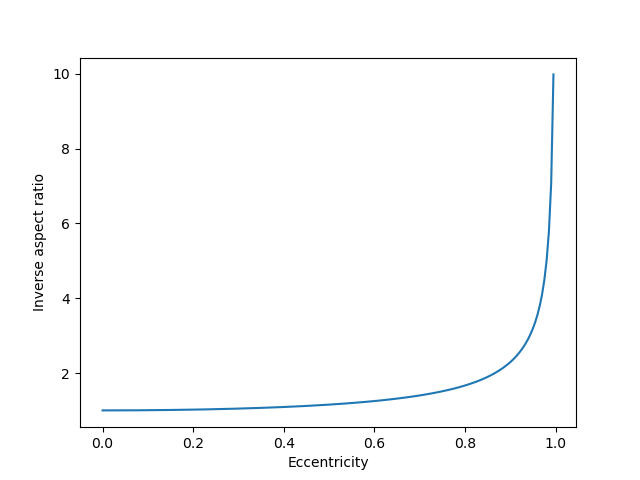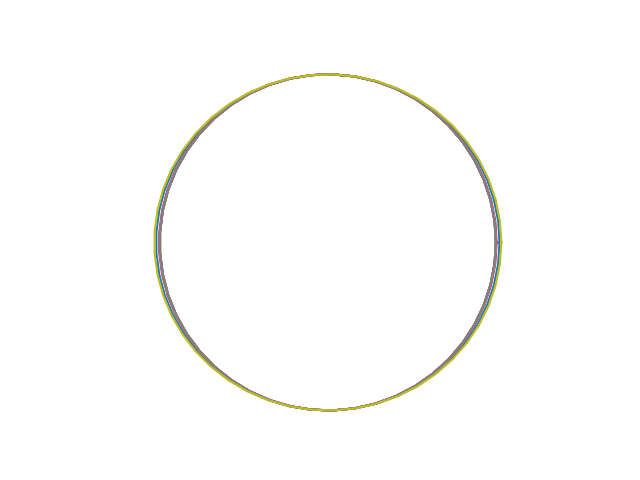# Eccentricity, Flattening, and Aspect Ratio

There are at least three common ways to describe the shape of an ellipse: eccentricity e, flattening f, and aspect ratio r. Each is a number between 0 and 1. (Flattening is also called ellipticity, which is a descriptive name, but unfortunately it sounds a lot like eccentricity.)

Although converting between these three descriptions is simple, it’s also a little counterintuitive. In particular, moderately large values of eccentricity correspond to small values of flattening. The previous post looked at how similar the orbits of all the planets are when you plot them all on the same scale. This is true even though the eccentricity of Venus is essentially 0 and the eccentricity of Pluto is 0.25.

Let a be the semi-major axis of the ellipse, the distance from the center to the furthest point of the ellipse.

Let b be the semi-minor axis of the ellipse, the distance from the center to the closest point on the ellipse.

Then the aspect ratio, flattening, and eccentricity are defined as follows:If we plot the orbits of all the planets, scaled so that b = 1 for all planets, then the distance to the furthest point in the orbit is 1/r. Here’s a graph of how 1/r varies as a function of e.Notice that 1/r hardly changes as e varies between, say, 0 and 0.4. So saying that Pluto has a “highly elliptical” orbit with e = 0.25 does not mean that the shape of its orbit is far from a circle. Here’s a plot of all the planet orbits in our solar system, or all the planet orbits plus the orbit of Pluto if you’d like to be pedantic.## Converting between eccentricity, flattening, and aspect ratio

I made the comment on Twitter that the orbit of the earth around the sun is closer to being an exact circle than the lines of constant longitude are. This means that the fact that the earth’s equatorial bulge is more geometrically significant than the elliptical nature of our orbit.

If you want to confirm this statement, you’ll need to know the shape of earth’s orbit and the shape of a meridian. But you’re most likely to find the former described in terms of eccentricity and the latter in terms of flattening. This is an example of why you might want to convert between different ways of describing the shape of an ellipse. Here are the conversion formulas.According to Wikipedia, the eccentricity of earth’s orbit is 0.01671 and the flattening is 1/298.3. To put these on a common scale, meridians have eccentricity: 0.08181, about five times the eccentricity of earth’s orbit.

Similarly, the earth’s orbit has flattening 0.00013962 or about 24 times the flattening of the meridians.

So you could say earth’s orbit is 4 times or 24 times closer to being a circle than earth’s meridians are. The two ratios are very different because the conversion between eccentricity and flattening is highly nonlinear.